# C# Arithmetic Operators | arithmetic operators in c# with example - c# - c# tutorial - c# netC# Arithemetic Operators

## What is Arithmetic Operator in C# ?

• Arithmetic operators take numerical values (either literals or variables) as their operands and return a single numerical value.
•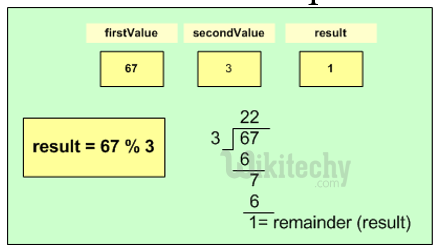• In C#-Programming the Arithmetic operators are used to perform mathematical calculations such as,Operator Description Example
+ Adds two operands A + B = 30
- Subtracts second operand from the first A - B = -10
* Multiplies both operands A * B = 200
/ Divides numerator by de-numerator B / A = 2
% Modulus Operator and remainder of after an integer division B % A = 0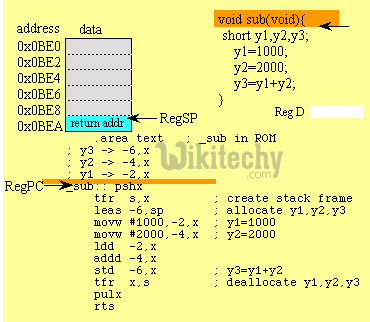## Code Explanation: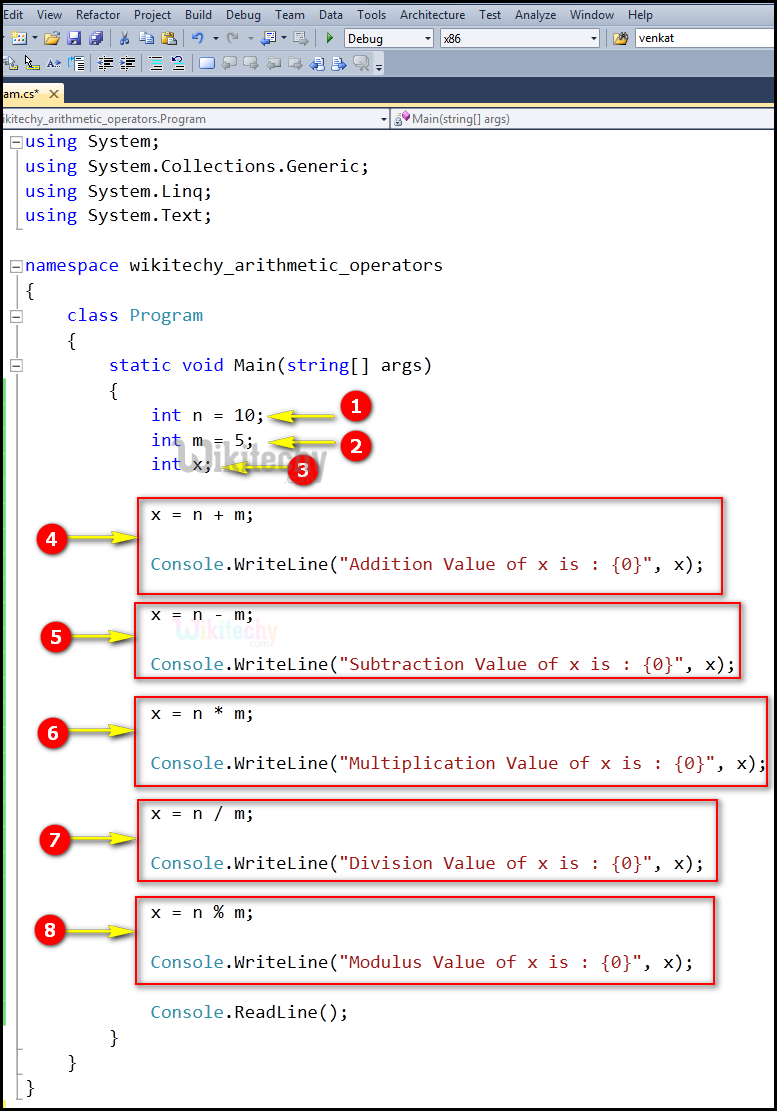1. Here we declare the integer variables n and assigning the value of the variable n=10. and m=5.
2. Here we declare another integer variables m and assigning the value of the variable m=5.
3. Here int x; is an integer variable.
4. In this statement, we perform an arithmetic operation for the variable "n" and "m" and the output value will be stored in the variable "x". And Console.WriteLine, the Main method specifies its behavior with the statement "Addition Value of x is:" to be displayed on the screen.
5. In this statement, we perform a subtraction operation for the variable "n" and "m" and the output value will be stored in the variable "x". And Console.WriteLine, the Main method specifies its behavior with the statement "Subtraction Value of x is:" to be displayed on the screen.
6. In this statement, we perform a multiplication operation for the variable "n" and "m" and the output value will be stored in the variable "x". And Console.WriteLine, the Main method specifies its behavior with the statement "Multiplication Value of x is:" to be displayed on the screen.
7. In this statement, we perform a division operation for the variable "n" and "m" and the output value will be stored in the variable "x". And Console.WriteLine, the Main method specifies its behavior with the statement "Division Value of x is:" to be displayed on the screen.
8. In this statement, we perform a mod operation for the variable "n" and "m" and the output value will be stored in the variable "x". Console.WriteLine, the Main method specifies its behavior with the statement " Modulus Value of x is:" to be displayed on the screen

## Sample C# examples - Output :1. Here the arithmetic operation for the variable "n" (value=10) and "m" (value=5) has been shown with its output result as "Addition Value of x is: 15".
2. Here the subtraction operation for the variable "n" (value=10) and "m" (value=5) has been shown with its output result as "Subtraction Value of x is: 5".
3. multiplication operation for the variable "n" (value=10) and "m" (value=5) has been shown with its output result as "Subtraction Value of x is: 50".
4. division operation for the variable "an" (value=10) and "m" (value=5) has been shown with its output result as "Division Value of x is: 2".
5. mod operation for the variable "n" (value=10) and "m" (value=5) has been shown with its output result as "Division Value of x is: 0".

## Basic Arithmetic Operations with strings :

• Plus (+) with string Identifiers
• Concatenates operand2 onto end of operand1
•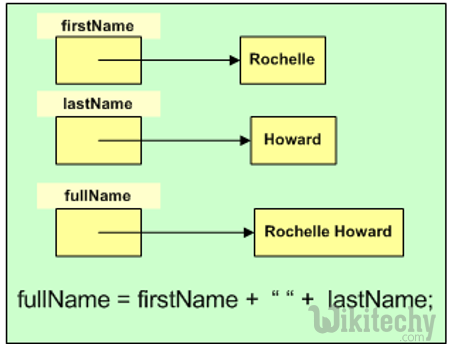## c# compound arithmetic operator :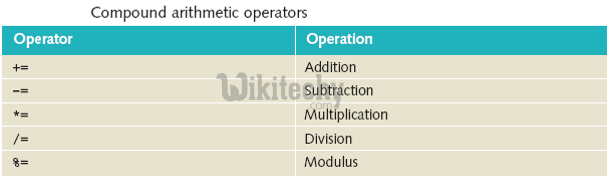## c# operator precedence :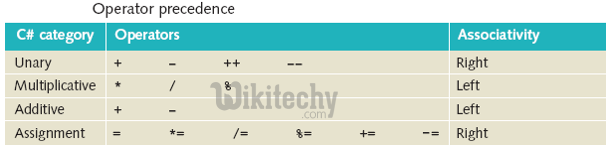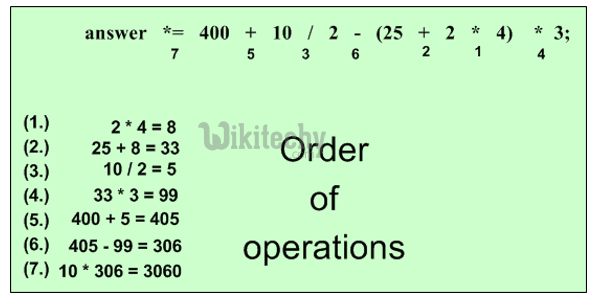## Operators in C# :

• Unary – take one operand
• Binary – take two operands
• Ternary (?:) – takes three operands